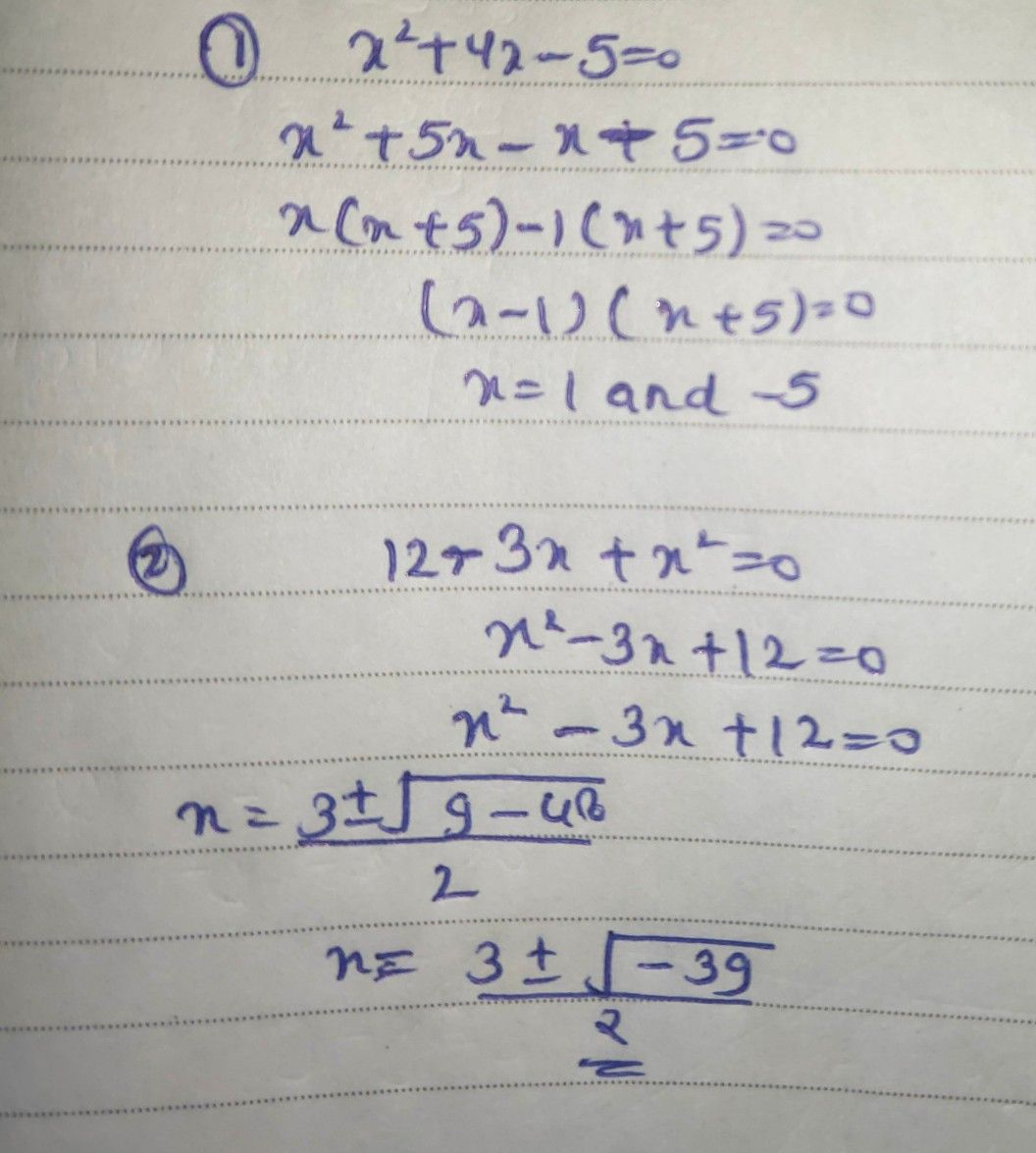Symbol
ProblemActivity $1.3:$ QI Builder... $Directions$ Transform the following mathematical sentences into quadratie inequalities by changing or adding only one element from the given. $x^{2}+4x-5=0$ $6$ $15-2x=x^{2}$ $1.$ $2$ $12-3x+x^{2}=0$ $7$ $\left(b^{2}-1\right)\left(2b+5\right)\leq 0$ $8$ $g^{2}\left(g+7\right)>8$ 3. $4t^{3}-7t+1\dfrac {\geq } {2}^{0}$ $x^{2}+10x<x$ $-$ $9$ $2h+5\geq h$ $3$ $4$ $5$ 5. $\left(a+4\right)\left(a+3\right)=5$ $10.3m+20>0$
7th-9th grade
Algebra
Search count: 106
SolutionQanda teacher - Askhina